# Chapter 21 Surface Area and Volumes of a Sphere RD Sharma Solutions Exercise 21.1 Class 9 Maths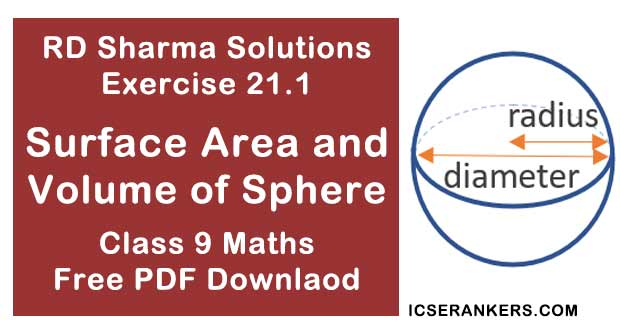Chapter Name RD Sharma Chapter 21 Surface Area and Volumes of a Sphere Exercise 21.1 Book Name RD Sharma Mathematics for Class 10 Other Exercises Exercise 21.2 Related Study NCERT Solutions for Class 10 Maths

### Exercise 21.1 Solutions

1. Find the surface area of a sphere of radius :

(i) 10.5 cm

(ii) 5.6 cm

(iii) 14 cm

Solution

(i) Given radius = 10.5 cm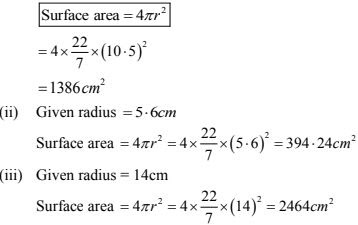2. Find the surface area of a sphere of diameter:
(i) 14 cm
(ii) 21 cm
(iii) 3.5 cm
Solution
(i) Diameter = 14 cm
Radius  = Diameter/2 = 14/2 = 7 cm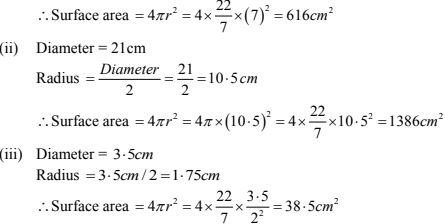3. Find the total surface area of a hemisphere and a solid hemisphere each of radius 10 cm. (Use π = 3.14)
Solution
The surface area of the hemisphere = 2πr2 .
= 2 × 3.14 × (10)2 = 628 cm2
The surface area of solid hemisphere = 3πr2
= 3 × 3.14 × (10)2 = 942 cm2

4. The surface area of a sphere is 5544 cm2 , find its diameter.
Solution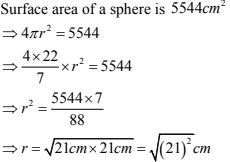⇒ r = 21 cm.
= 2(21 cm)
= 42 cm.

5. A hemispherical bowl made of brass has inner diameter 10.5 cm. Find the cost of tin - plating it on the inside at the rate of Rs. 4 per 100 cm2.
Solution
Given
Inner diameter of hemisphere bowl = 10.5 cm
Radius = 10.5/2 cm = 5.25 cm
Surface area of hemispherical bowl = 2πr
= 2[22/7] × (5.25)2 cm2
= 173.25 cm2
Cost of tin planning 100 cm2 area = Rs. 4
Cost of tin planning 173.25 cm2 area = Rs.[(4 × 173.25)/100] = Rs. 6.93
Thus, The cost of tin plating the inner side of hemisphere bowl is Rs. 6.93

6. The dome of a building is in the form of a hemisphere. Its radius is 63 dm. Find the cost of painting it at the rate of Rs. 2 per sq. m.
Solution
Dome Radius = 63 d m = 6.3 m
Inner S.A of dome = 2πr2 = 2 × 22/7 × (6.3)2 = 249.48 m2
Now, cost of 1 m2  = Rs. 2
∴ Cost of 249. 48 m2  = Rs. [2×249.48]
= Rs. 498.96

7. Assuming the earth to be a sphere of radius 6370 km, how many square kilo metres is area of the land, if three - fourth of the earth's surface is covered by water ?
Solution
3/4 th of earth surface is covered by water
∴ 1/4 th earth surface is covered by land
∴ Surface area covered by land = 1/4 × 4πr2
= 1/4 × 4 × 22/7 × (6370)2
= 127527.4 km2

8. A cylinder of same height and radius is placed on the top of a hemisphere. Find the curved surface area of the shape if the length of the shape be 7 cm.
Solution
Given length of the shape = 7 cm
But length = r + r
⇒ 2r = 7 cm
⇒ r = 7/2 cm
⇒ r = 3.5 cm
Also; h = r
Total S.A of shape = 2πrh + 2πr2 = 2πr ×r + 2πr2
= 2πr2 + 2πr2
=4πr2
= 4 × 22/7 × (3.5)2
= 154 cm2

9. A wooden toy is in the form of a cone surmounted on a hemisphere. The diameter of the base of the cone is 16 cm and its height is 15 cm. Find the cost of painting the toy at Rs. 7 per 100 cm2 .
Solution
Diameter of cone = 16 cm
∴ Radius of cone  8 cm
Height of cone = 15 cm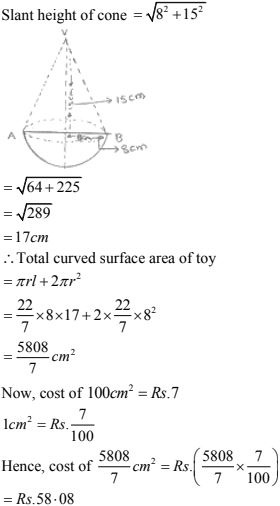10. A storage tank consists of a circular cylinder with a hemisphere adjoined on either end. If the external diameter of the cylinder be 1.4 m and its length be 8 m, find the cost of painting it on the outside at the rate of Rs. 10 per m2 .
Solution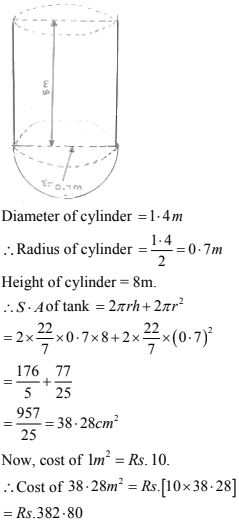11. The diameter of the moon is approximately one fourth of the diameter of the earth. Find the
ratio of their surface areas.
Solution
Let the diameter of the earth is d then, diameter of moon will be d/4
Radius of moon = (d/2)/4 = d/8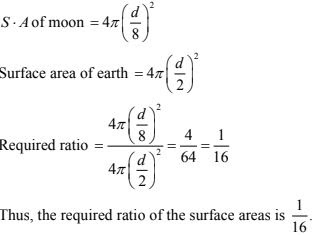12. A hemi-spherical dome of a building needs to be painted. If the circumference of the base of the dome is 17.6 cm, find the cost of painting it, given the cost of painting is Rs. 5 per l00 cm2 .
Solution
Given that only the rounded surface of the dome to be painted, we would need to find the curved surface area of the hemisphere to know the extent of painting that needs to be done.
Now, circumference of the dome = 17.6 m.
Therefore, 17.6 = 2πr
2 ×22/7 × r = 17.6 m.
So, the radius of the dome = 17.6 × 7/(2×22) = 2.8m
The curved surface area of the dome = 2πr2
= 2 × 22/7 × 2.8 × 2.8 cm2 =49.28 m2
Now, cost of painting 100 cm2 is Rs. 5.
So, cost of painting 1m2 = Rs. 500
Therefore, cost of painting the whole dome
= Rs. 500 × 49.28 = Rs. 24640

13. The front compound wall of a house is decorated by wooden spheres of diameter 21 cm, placed in small supports as shown in Fig. below. Eight such spheres are used for this purpose, and are to be painted silver. Each support is a cylinder of radius 1 .5 cm and height 7 cm and is to be painted black. Find the cost of paint required if silver paint costs 25 paise per cm2  and black paint costs 5 paise per cm2 .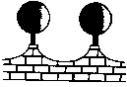Solution
Wooden sphere radius= (21/2) cm = 10.5 cm
Surface area of a wooden sphere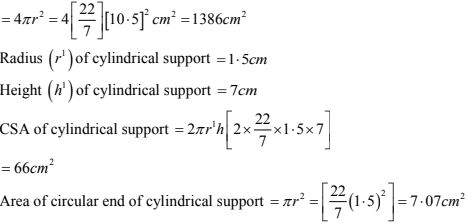= 66 cm2
Area of circular end of cylindrical support = πr2 = [22/7(1.5)2 ] = 7.07 cm2
Area to be painted silver = [8 × (1386 - 7.07)] cm2
= 8(1378.93) cm2
= 11031.44 cm2
Cost occurred in painting silver color
= Rs. (11031.44 × 0.25) = Rs. 2757.86
Area to painted black = (8 × 66) cm2 = 528 cm2
Cost occurred in painting black color = Rs. (528 × 0.05) = Rs. 26.40
∴ Total cost occurred in painting  = Rs. (2757.86 + 26.40) = Rs. 2784.26